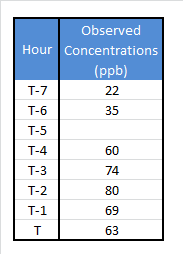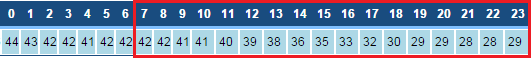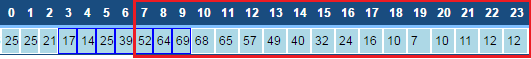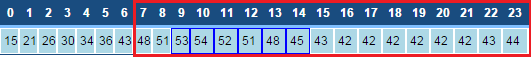# AirNow Updates to Ozone Real-time Reporting and Daily Reporting

On 4/21/16, the AirNow Data Management Center rolled out two new changes which effect how ozone is reported.

# Ozone NowCast

The ozone NowCast replaces the ozone surrogate (slope/intercept method) for real time reporting. The ozone NowCast calculation is similar to the PM2.5 and PM10 NowCast calculations described in this presentation, but does include some slight differences (indicated by the bold text below). The process for calculating the ozone NowCast is as follows:

1. Compute the concentrations range (max-min) over the last 8 hours.

2. Divide the range by the maximum concentration in the 8 hour period to obtain the scaled rate of change.

3. Compute the weight factor by subtracting the scaled rate from 1. There is no minimum weight factor (PM2.5 and PM10 have a minimum weight factor of 0.5). There is a maximum weight factor of 1.

4. Multiply each hourly concentrations by the weight factor raised to the power of how many hours ago the concentration was measured (for the current hour, the factor is raised to the zero power).

5. Compute the NowCast by summing these products and dividing by the sum of the weight factors raised to the power of how many hours ago the concentration was measured.

Missing data is handled the same way as the PM2.5 and PM10 NowCast: 2 of the last 3 hours of data must be valid for a NowCast calculation. An example of a NowCast calculation is below, with a missing hour included.

### Example Calculation1. Range: 80-22=58
2. Scaled rate of change: 58/80= .725
3. Weight Factor: 1-.725= .275
4. 63*(.275)0+69*(.275)1+80*(.275)2+74*(.275)3+60*(.275)4+Missing*(.275)5+35*(.275)6+22*(.275)7= 89.925
5. 89.925/ (.275)0+(.275)1+(.275)2+(.275)3+(.275)4+(.275)5+(.275)6+(.275)7=

# Daily maximum 8-hour average ozone

In accordance with the 2016 National Ambient Air Quality Standard (NAAQS), AirNow-Tech has updated the hours considered in a daily maximum 8-hour average ozone calculation. The change is as follows:

"The daily maximum 8-hour average ozone concentration for a given day is the highest of the 17 consecutive 8-hour averages beginning with the 8-hour period from 7:00 a.m. to 3:00 p.m. Local Standard Time (LST) and ending with the 8-hour period from 11:00 p.m. to 7:00 a.m. LST the following day (i.e., the 8-hour averages for 7:00 a.m. to 11:00 p.m.LST).

A daily maximum 8-hour average Ozone concentration shall be considered valid if valid 8-hour averages are available for at least 13 of the 17 consecutive 8-hour periods starting from 7:00 a.m. to 11:00 p.m. LST. In addition, in the event that fewer than 13/17 valid 8-hour averages are available, a daily maximum 8-hour average ozone concentration shall also be considered valid if it is greater than the level of the NAAQS"

The purpose of this change was to avoid double counting overnight exceedances from a single ‘event’ (e.g. 73 ppb 8-hour average at 11:00 p.m. and 12:00 a.m. counting as two exceedances). Please note that, as before, 6/8 hours are needed for a valid 8-hour average (75%).

Below are possible changes and scenarios that you can expect to see:

1. Changes in daily maximum 8-hour average if the maximum 8-hour average occured between 00:00 and 06:00

2. A day that did not meet 75% completeness criteria before (<18/24 8-hour averages in a day) is now valid (>=13/17 8-hour averages from 07:00 to 23:00)

3. A day that did meet 75% completeness criteria before (>=18/24 8-hour averages in a day) is now invalid (<13/17 8-hour averages from 07:00 to 23:00)

An example of each of these cases is below:

### Morning Max

Below are hourly 8-hour averages for a given day.Old daily maximum 8-hour average: 44 ppb
New daily maximum 8-hour average: 42 ppb

### Invalid to Valid

Below are hourly 8-hour averages for a given day. The blue outline indicates insufficient data/invalid 8-hour averagesOld # of valid 8-hour averages: 17/24 (<75%) Old daily maximum 8-hour average: Insufficient data

New # of valid 8-hour averages: 14/17 (>=75%)
New daily maximum 8-hour average: 68 ppb

### Valid to Invalid

Below are hourly 8-hour averages for a given day. The blue outline indicates insufficient data/invalid 8-hour averagesOld # of valid 8-hour averages: 18/24 (>=75%)
Old daily maximum 8-hour average: 51 ppb

New # of valid 8-hour averages: 11/17 (<75%)
New daily maximum 8-hour average: Insufficient data

Hi Mwhylton . . . Are there any plots of ozone data similar to those in the presentation on PM? Or are there data available for actual hourly values, 8-hr averages, and the new Reff numbers? I would like to understand what this change looks like with live data. Thank you!

Mark, we have nothing official on hand here at the AirNow DMC that is similar to what is shown in the PM2.5 NowCast presentation. I’ve asked the EPA if they have any presentations/analysis available on the ozone NowCast that they could pass along. If they do not, we would likely be able to create something similar.

-Marcus

Here are some plots for the new ozone NowCast:

• OldNowcast: Surrogate (slope/intercept) method used prior to the ozone NowCast
• NewNowcast: based on a 4-hour average Not used
• NewNowcast_8hr: based on an 8-hour average.This is the implemented NowCast
• Mean1Hr: 1-hour observations
• Mean8Hr: end 8-hour averages (e.g. for 12:00, the 8-hour average is from 5:00-12:00, not 12:00-19:00)